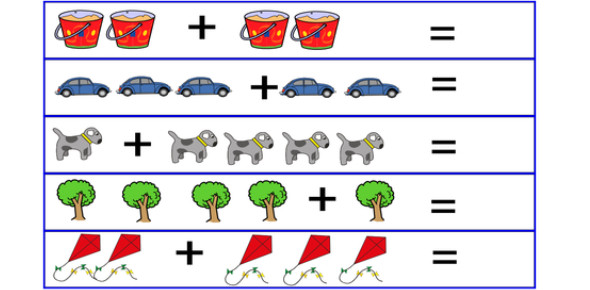Simple Addition Quiz For Kids: Trivia

10 Questions | Total Attempts: 4974SettingsWould you be able to teach simple addition for kids? Do you think you can help them pass this quiz? Addition is an essential skill to develop for kids when they are in school. It is not only helpful for future math classes, but math is relevant in our everyday lives. Practice helps kids become better at math. Take this quiz and see how it all adds up.

• 1.
8 + 6 =?
• A.

11

• B.

12

• C.

13

• D.

14

• 2.
• A.

10

• B.

11

• C.

12

• D.

13

• 3.
5 + 5 = ?
• A.

11

• B.

12

• C.

10

• D.

9

• 4.
Use the line number to solve  2+ 8 =?
• A.

11

• B.

10

• C.

12

• D.

9

• 5.
9 + 5 = ?
• A.

12

• B.

13

• C.

14

• D.

16

• 6.
• A.

66

• B.

76

• C.

56

• D.

86

• 7.
8 + 8 =?
• A.

17

• B.

16

• C.

15

• D.

14

• 8.
Partitioning 10 tens + 5 ones = ?
• A.

10

• B.

15

• C.

5

• D.

11

• 9.
5 + 3 = ?
• A.

8

• B.

6

• C.

9

• D.

7

• 10.
• A.

-

• B.

=

• C.

+

• D.

/

Related TopicsBack to top